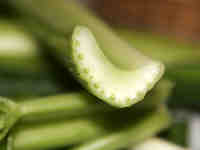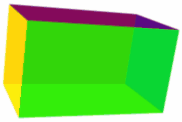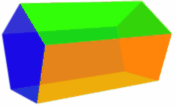# Cross Sections

A cross section is the shape we get when cutting straight through an object.The cross section of this object is a triangle.

It is like a view into the inside of something made by cutting through it.

 This is a cross-section of a piece of celery.## Geometry

In geometry it is the shape made when a solid is cut through by a plane.### Example:

The cross section of this circular cylinder is a circle

We don't draw the rest of the object, just the shape made when you cut through.### Example:

The cross section of a rectangular pyramid is a rectangle

Cross sections are usually parallel to the base like above, but can be in any direction.

### Example:The vertical cross section through the center of this torus is two circles!And the horizontal cross section is an annulus

## More Examples of Cross Sections

 Square Prism: Cross-Section:Cube: Cross-Section:Pentagonal Prism: Cross-Section: